# 'How many turns should I make to completely shuffle the surface of the Rubik's cube?' Is a very difficult problem in mathematics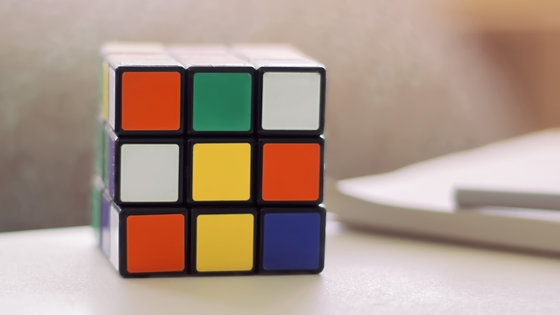Popular throughout the world, ``

Rubik's Cube '' is a three-dimensional puzzle that is difficult enough for beginners to align one side, but the world's fastest record that aligns all six sides has shrunk to about 4 seconds , depending on experienced players Research is being conducted every day. However, according to Tim Garoni, an associate professor at the Department of Mathematics at Monash University , it is more difficult in the mathematical world to completely shuffle the surface of a Rubik's cube than to align it.

How hard is it to scramble Rubik's Cube?
https://theconversation.com/how-hard-is-it-to-scramble-rubiks-cube-129916

Many mathematicians have studied 'shuffle to create randomness'. Dave Bayer Professor mathematician of persistent-Daiakonisu professor and Columbia University, is a mathematician at Stanford University, a research paper on 'Riffle Shuffle' in 1990 announced we are. Rifle shuffle is a way to shuffle cards like the following movie, Professor Diaconis and Professor Bayer said, `` It is necessary to repeat riffle shuffle seven times to make the order of cards completely random '' Prove that.

Cardistry for Beginners: Shuffles-Riffle Shuffle Tutorial-YouTube

Also, you can find out what happens if you stir the cards on your desk by reading the following article.

There is a mathematician who challenges with gachinko to `` How many seconds will you mix perfectly if you stir the card on the desk ''-GIGAZINE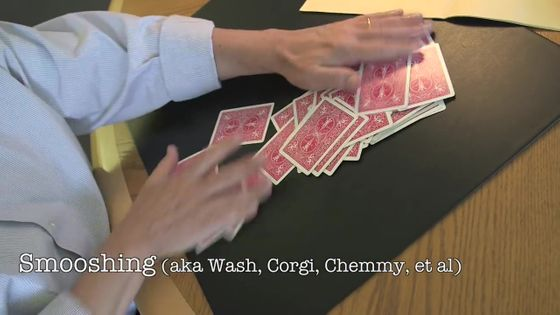There are as many as 4325 Kyo 2003 trillion 247,489,866,000 patterns of Rubik's cube, but in 2010 it was proved that `` Rubik's cube can always solve any pattern within just 20 moves '' Was. The number '20 hands' is called 'God's number' in the Rubik's cube world. On the other hand, 'How much work is needed to randomly move a Rubik's cube to a completely shuffled state is a very difficult problem,' says Garoni.

The Rubik's Cube can rotate one of the three rows in the X, Y, or Z axis by 90, 180, or 270 degrees. In order to shuffle the surface of the Rubik's Cube, it is necessary to repeat the act of randomly performing one of 15 operations (the first time, 18 operations). According to Garoni, `` to completely shuffle the Rubik's cube '' is mathematically described as `` the probability of occurrence of all patterns approaches as close as 1 / 43,325K 2003,274,488,9856,000 It means that this state is called 'probability distribution is uniform'.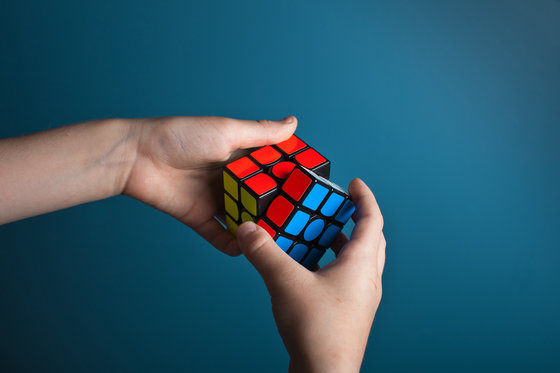According to Garoni, the operation of the Rubik's cube can be explained by a stochastic model called '

Markov chain '. And ' how much operation is needed to make the probability distribution in the Rubik's cube as infinite as possible ' can be confirmed by the ' Markov chain Monte Carlo method ' that samples the probability distribution with an algorithm. However, performing the Markov chain Monte Carlo method with a general Rubik's cube of 3 blocks × 3 blocks × 3 blocks requires too much calculation, so Garoni says that it is an unsolved problem at the time of article creation Professor says.

Therefore, Associate Professor Galoni uses a 'Pocket Cube', a 2-block x 2-block x 2-block Rubik's cube. The total number of pocket cube patterns is 3,674,160, and the number of Gods is 11.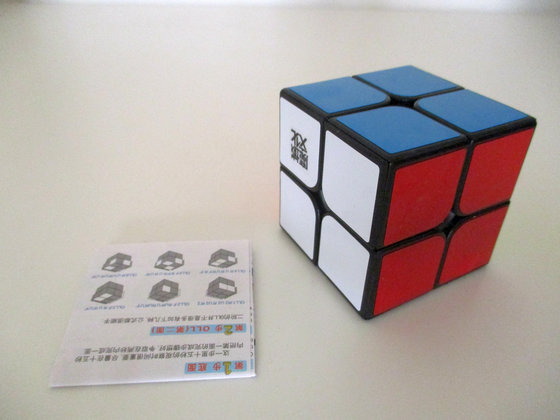by Abdulla Al Muhairi

The following graph shows the probability distribution of a pocket cube based on a simulation. T on the horizontal axis is the number of steps, and d (t) on the vertical axis means `` how far away from the uniform probability distribution '', and the smaller the d (t), the more `` shuffled '' Indicates that According to Garoni, the line that is generally 'well shuffled' is when d (t) is below 0.25, and for pocket cubes when t is 19. In other words, if you do not operate at least 19 times, it can not be mathematically said that 'the pocket cube was shuffled firmly'.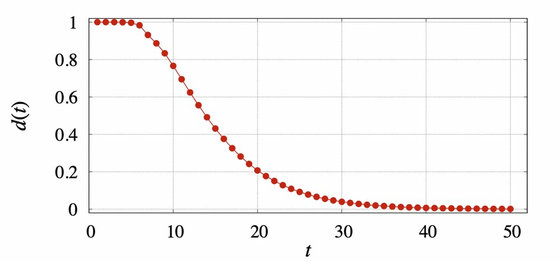Also, as the number of moves increases, the probability distribution becomes more uniform. It seems that d (t) is 0.092 when operated 25 times, 0.0012 when operated 50 times, and 0.00000017 when operated 100 times.

The Markov chain Monte Carlo algorithm for Pocket Cube is published on GitHub .

in Science,   Video, Posted by log1i_yk